## General Question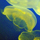# Engineering Piston Question?

Asked by jdstevens (4) July 29th, 2009

I have a gas spring rod. One side of the piston is pressurized with nitrogen gas while the other side is air at atmospheric pressure. If you apply a constant axial force, how do you find the time it takes to move the piston a given amount in the direction that compresses the nitrogen. I am really looking for the formula to use to calculate this.

Observing members: 0Composing members: 0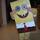I don’t believe you have enough information to figure this out. Normally those pistons provide damping that slows the action down. A gas charge by itself would make the piston move very rapidly so damping must be added. The damping properties need to be known.

itsjustmatt (86)“Great Answer” (1) Flag as…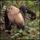@itsjustmatt: Assume a frictionless spherical cow :-)

barumonkey (1069)“Great Answer” (1) Flag as…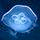Assuming the piston is rigid, chamber is sealed and atmospheric pressure> nitrogen pressure

You know the piston area, therefore you know the force acting on both sides of the piston. Force = Pressure X Area. You know atmospheric pressure. Assuming nitrogen is an ideal gas. Using P=rho.R.T you can calcualte the pressure of nitrogen gas and find the force exerted by nitrogen gas on the piston

Newton’s Law, F=MA
U know the resultant force which is basically Atmospheric force- nitrogen force. U must know the mass of the piston. THerefore you can calcualte the acceleration of the piston
U know that a= (Final velocity- Initial velocty)/ Time.

The final velocity will be zero because the piston will cease to move once the force is equal on both sides. You got to find the initial velocity to find the time needed to compress the nitrogen gas. I am stucked here.

mea05key (1812)“Great Answer” (4) Flag as…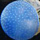The “constant axial force” is a bit ambiguous, because it seems you mean it suddenly is applied then it’s constant. In that case, without damping, which as @itsjustmatt points out is not mentioned, the system is elastic and will oscillate like a spring.

dabbler (18865)“Great Answer” (0) Flag as…or